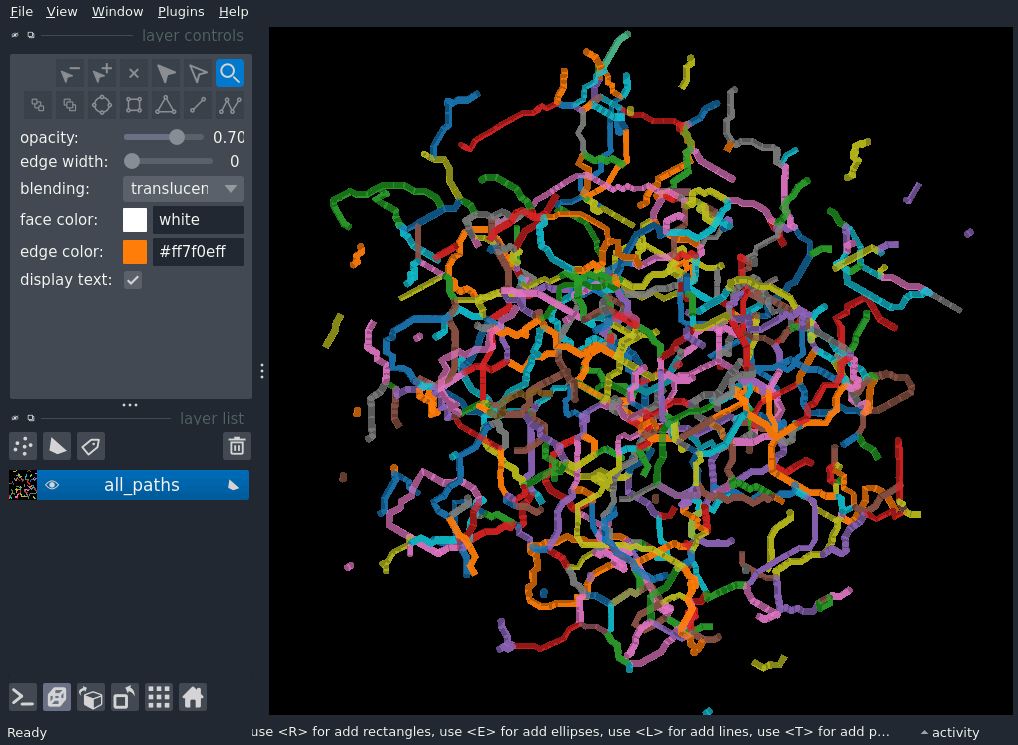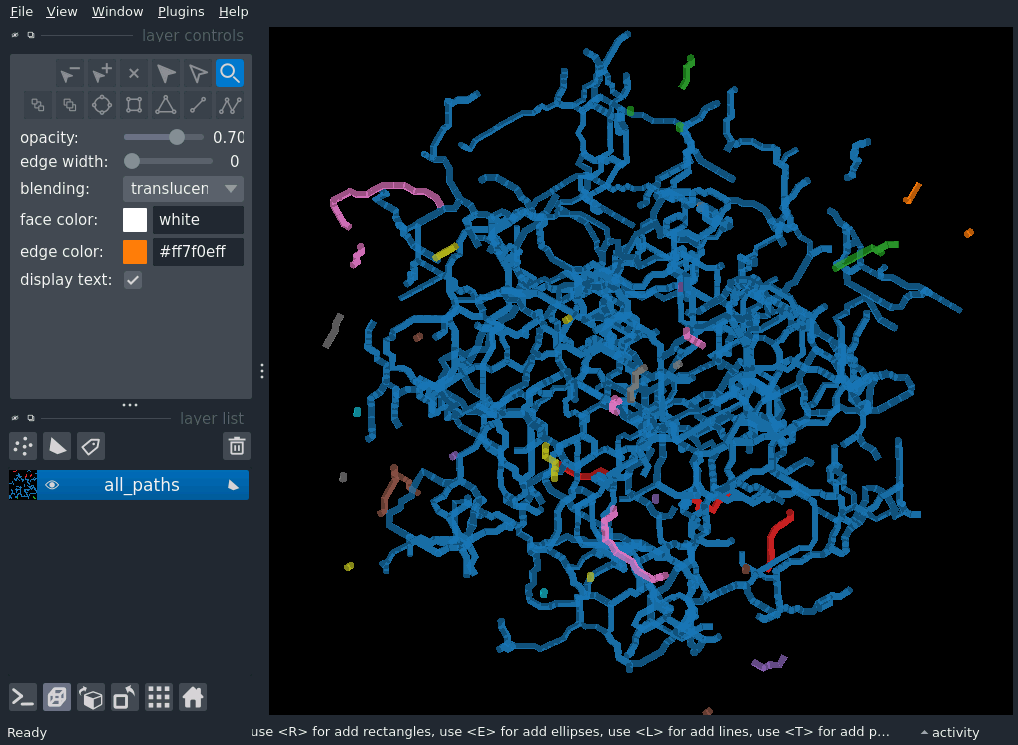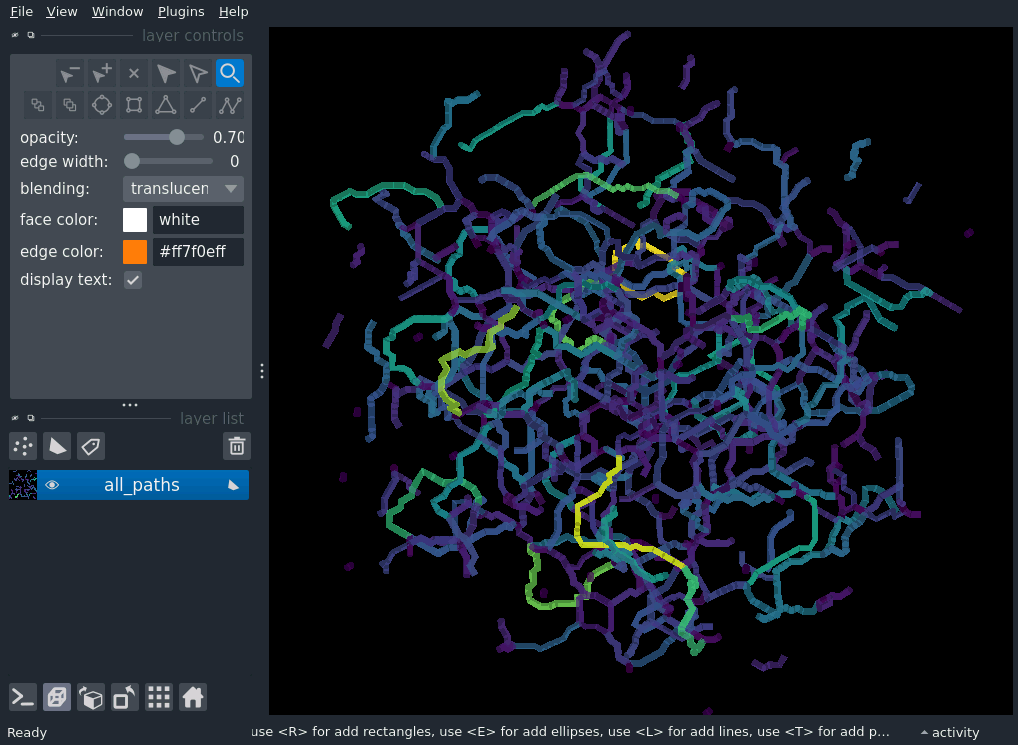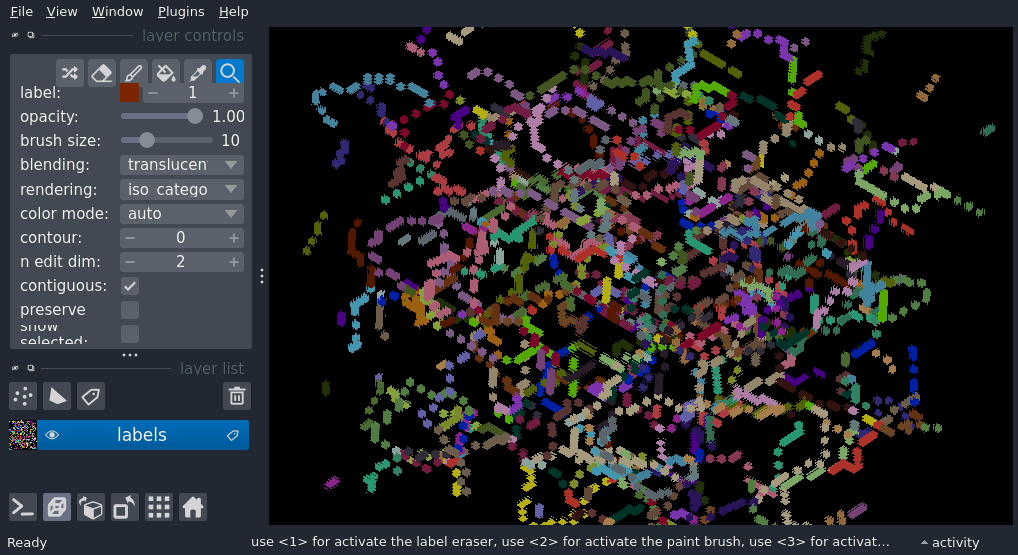# Display 3D skeletons in napari#

There are two ways to display 3D skeletons in napari. First, we demonstrate how to use the napari Shapes layer to draw Paths for each skeleton path. As of napari 0.4.14, however, 3D interactivity in the Shapes layer is not ideal, so we also demonstrate how to use the Labels layer.

## Using the Shapes layer#

The napari Shapes layer allows displaying of 2D and 3D paths, and coloring them by tabular properties, which makes it ideal for displaying skeletons, and the measurements provided by skan.

```import napari
import numpy as np
import skan
from skimage.data import binary_blobs
from skimage.morphology import skeletonize
import scipy.ndimage as ndi
```

Note

We use synthetic data here. If you have a cool 3D skeleton dataset that you’d like to show off in these docs, let us know!

```blobs = binary_blobs(64, volume_fraction=0.3, n_dim=3)
binary_skeleton = skeletonize(blobs)
skeleton = skan.Skeleton(binary_skeleton)
```
```all_paths = [
skeleton.path_coordinates(i)
for i in range(skeleton.n_paths)
]
```
```paths_table = skan.summarize(skeleton)
```
```paths_table['path-id'] = np.arange(skeleton.n_paths)
```

First, we color by random path ID, showing each path in a distinct color using the matplotlib “tab10” qualitative palette. (Coloring by path ID directly results in “bands” of nearby paths receiving the same color.)

```paths_table['random-path-id'] = np.random.default_rng().permutation(skeleton.n_paths)
```
```viewer = napari.Viewer(ndisplay=3)

all_paths,
shape_type='path',
properties=paths_table,
edge_width=0.5,
edge_color='random-path-id',
edge_colormap='tab10',
)
```
```napari.manifest -> 'skan' could not be imported: Could not find file 'napari.yaml' in module 'skan'
```We can also demonstrate that most of these branches are in one skeleton, with a few stragglers around the edges, by coloring by skeleton ID:

```skeleton_layer.edge_color = 'skeleton-id'
# for now, we need to set the face color as well
skeleton_layer.face_color = 'skeleton-id'
```Finally, we can color the paths by a numerical property, such as their length.

```skeleton_layer.edge_color = 'branch-distance'
skeleton_layer.edge_colormap = 'viridis'
# for now, we need to set the face color as well
skeleton_layer.face_color = 'branch-distance'
skeleton_layer.face_colormap = 'viridis'
```## Using the Labels layer#

We can also visualize the pixels of the skeleton as a Labels layer, with each path ID appearing as a different label. The downside with this approach is that junction pixels are arbitrarily assigned to one of the branches incident on that junction. Therefore, removing that branch would cause the junction pixel to be removed, which could incorrectly disconnect the skeleton at that point.

However, the rich 3D interactivity of the labels layer does allow prunning of branches in 3D, which could be extremely useful for manual curation of the skeleton, as long as propert care is taken in downstream processing of the edits.

```labels = np.asarray(skeleton)

viewer2 = napari.view_labels(
labels,
properties=paths_table,
opacity=1,
ndisplay=3,
)
```